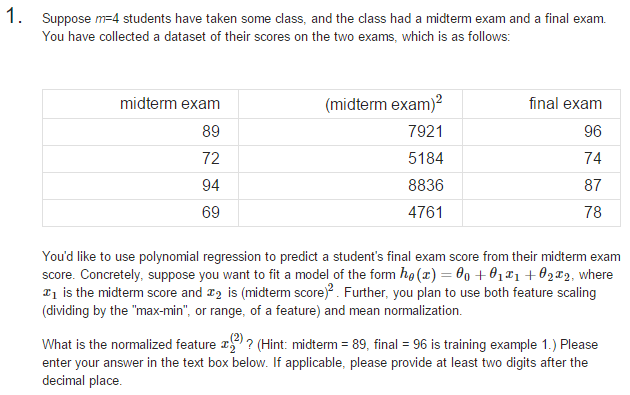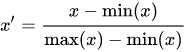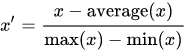2 views

This has become quite a frustrating question, but I've asked in the Coursera discussions and they won't help. Below is the question:I've gotten it wrong 6 times now. How do I normalize the feature? Hints are all I'm asking for.

I'm assuming x_2^(2) is the value 5184 unless I am adding the x_0 column of 1's, which they don't mention but he certainly mentions in the lectures when talking about creating the design matrix X. In which case, x_2^(2) would be the value 72. Assuming one or the other is right (I'm playing a guessing game), what should I use to normalize it? He talks about 3 different ways to normalize in the lectures: one using the maximum value, another with the range/difference between max and mins, and another the standard deviation -- they want an answer correct to the hundredths. Which one am I to use? This is so confusing.

by (33.1k points)

Normalization: It is a technique often applied as part of data preparation for machine learning. The goal of normalization is to change the values of numeric columns in the dataset to a common scale, without distorting differences in the ranges of values.

For your problem, you can use

• Min-Max Normalization• Mean NormalizationWhere x is the original value.

For example:

from sklearn import preprocessing

std_scale = preprocessing.StandardScaler().fit(train_norm)

x_train_norm = std_scale.transform(train_norm)

x_test_norm = std_scale.transform(test_norm)

testing_norm_col = pd.DataFrame(x_test_norm, index=test_norm.index, columns=test_norm.columns)

x_test.update(testing_norm_col)